# JAC Class 9 Maths Solutions Chapter 6 Lines and Angles Ex 6.3

Jharkhand Board JAC Class 9 Maths Solutions Chapter 6 Lines and Angles Ex 6.3 Textbook Exercise Questions and Answers.

## JAC Board Class 9th Maths Solutions Chapter 6 Lines and Angles Ex 6.3

Page-107

Question 1.
In Fig, sides QP and RQ of ΔPQR are produced to points S and T respectively. If ∠SPR = 135° and ∠PQT = 110°, find ∠PRQ.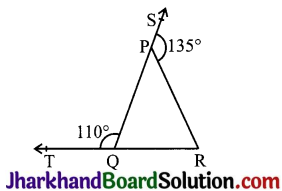Given, ∠SPR = 135° and ∠PQT = 110°
∠SPR + ∠QPR = 180° (SQ is a straight line)
⇒ 135° + ∠QPR = 180°
⇒ ∠QPR = 45°
Also, ∠PQT +∠PQR = 180° (TR is a straight line)
⇒ 110° + ∠PQR= 180°
⇒ ∠PQR = 70°
Now, ∠PQR + ∠QPR + ∠PRQ = 180°
(Sum of the interior angles of the triangle)
⇒ 70° + 45° + ∠PRQ = 180°
⇒ 115° + ∠PRQ = 180°
⇒ ∠PRQ = 65°

Question 2.
In Fig, ∠X = 62°, ∠XYZ = 54°. If YO and ZO are the bisectors of ∠XYZ and ∠XZY respectively of ΔXYZ, find ∠OZY and ∠YOZ.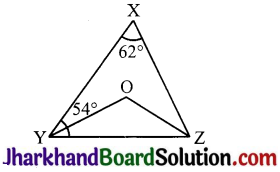Given ∠X = 62°, ∠XYZ = 54°
YO and ZO are the bisectors of ∠XYZ and ∠XZY respectively.
∠X + ∠XYZ + ∠XZY = 180° (Sum of the interior angles of the triangle)
⇒ 62° + 54° + ∠XZY = 180°
⇒ 116° + ∠XZY = 180°
⇒ ∠XZY = 64°

Now, ∠OZY = $$\frac {1}{2}$$ ∠XZY (ZO bisector.)
⇒ ∠OZY = 32°
also, ∠OYZ = $$\frac {1}{2}$$ ∠XYZ (YO is the bisector.)
⇒ ∠OYZ = 27°
Now, ∠OZY + ∠OYZ + ∠YOZ = 180° (Sum of the interior angles of the triangle)
⇒ 32° + 27° + ∠YOZ = 180°
⇒ 59° + ∠YOZ = 180°
⇒ ∠YOZ = 121°Question 3.
In, Fig , if AB || DE, ∠BAC = 35° and ∠CDE = 53° and ∠DCE.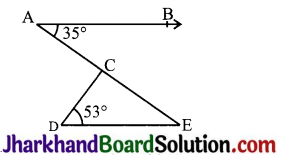Given, AB || DE, ∠BAC = 35° and ∠CDE = 53°
∠BAC = ∠CED (Alternate interior angles.)
∴ ∠CED = 35°
Now, ∠DCE + ∠CED + ∠CDE = 180° (Sum of the interior angles of the triangle)
⇒ ∠DCE + 35° + 53° = 180°
⇒ ∠DCE + 88° = 180°
⇒ ∠DCE = 92°

Question 4.
In Fig, if lines PQ and RS intersect at point T, such that ∠PRT = 40°, ∠RPT = 95° and ∠TSQ = 75°, find ∠SQT.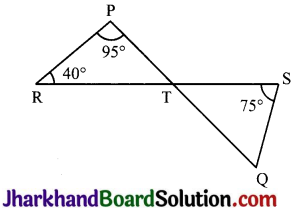Given, ∠PRT = 40°, ∠RPT = 95° and ∠TSQ = 75°
∠PRT + ∠RPT + ∠PTR = 180° (Sum of the interior angles of the triangle)
⇒ 40° + 95° + ∠PTR = 180°
⇒ 135° + ∠PTR = 180°
⇒ ∠PTR = 45°
∠STQ = ∠PTR = 45° (Vertically opposite angles)
Now, ∠TSQ + ∠STQ + ∠SQT = 180° (Sum of the interior angles of the triangle)
⇒ 75° + 45° + ∠SQT = 180°
⇒ 120° + ∠SQT = 180°
⇒ ∠SQT = 60°

Page-108

Question 5.
In Fig, if PQ || PS, PQ || SR, ∠SQR = 28° and ∠QRT = 65°, then find the values of x and y.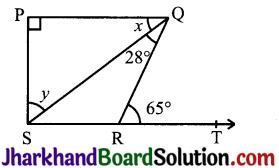Given, PQ || PS, PQ || SR, ∠SQR = 28° and ∠QRT = 65°
x + ∠SQR = ∠QRT (Alternate angles as QR is transversal)
⇒ x + 28° = 65°
⇒ x = 37°
also, ∠QSR = x (Alternate angles)
⇒ ∠QSR = 37°
also, ∠QRS+∠QRT=180°(Linear pair)
⇒ ∠QRS + 65° = 180°
⇒ ∠QRS =115°
Now, ∠P + ∠Q+ ∠R + ∠S = 360°
(Sum of the angles in a quadrilateral)
⇒ 90° + 65° + 115° + ∠S = 360°
⇒ 270° + y + ∠QSR = 360°
⇒ 270° + y + 37° = 360°
⇒ 307° + y = 360°
⇒ y = 53°Question 6.
In Fig, the side QR of APQR is produced to a point S. If the bisectors of ∠PQR and ∠PRS meet at point T, then prove that ∠QTR = $$\frac{1}{2}$$ ∠QPR.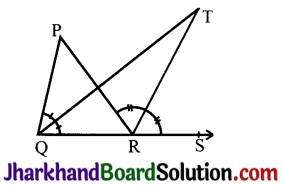To prove: ∠QTR = $$\frac{1}{2}$$ ∠QPR.
⇒ $$\frac{1}{2}$$ QPR = ∠TRS – ∠TQR …………(ii)
∠QTR = $$\frac{1}{2}$$ ∠QPR